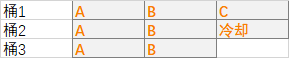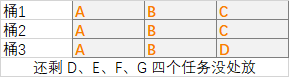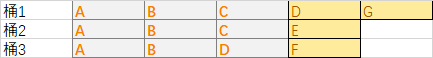# 力扣 621. 任务调度器

## 题目描述

输入：tasks = ["A","A","A","B","B","B"], n = 2

在本示例中，两个相同类型任务之间必须间隔长度为 n = 2 的冷却时间，而执行一个任务只需要一个单位时间，所以中间出现了（待命）状态。


输入：tasks = ["A","A","A","B","B","B"], n = 0

["A","A","A","B","B","B"]
["A","B","A","B","A","B"]
["B","B","B","A","A","A"]
...



输入：tasks = ["A","A","A","A","A","A","B","C","D","E","F","G"], n = 2

A -> B -> C -> A -> D -> E -> A -> F -> G -> A -> (待命) -> (待命) -> A -> (待命) -> (待命) -> A


• 1 <= task.length <= 104
• tasks[i] 是大写英文字母
• n 的取值范围为 [0, 100]

## 桶思想图解

• 冷却时间 n = 2
• 任务列表 tasks = [A, A, A, B, B, B, C]

• 只要同一个桶不出现相同的任务，就能够满足相同任务间隔至少为 n 的冷却条件。• (h - 1) * (n + 1) + w
• w 为最后一个桶的实际占用宽度，可以通过计算一共有几种任务达到了最大任务数 h 得出。本例中，AB 都达到了最大任务数，所以 w == 2
• 代入计算，最后答案为 8

• [A, B, C, A, B, 冷却, A, B]

• 冷却时间还是为 n = 2
• 任务列表增加了一些任务 tasks = [A, A, A, B, B, B, C, C, D, D, E, F, G]• len(tasks)

• [A, B, C, D, G, A, B, C, E, A, B, D, F]

• max(len(tasks), (h - 1) * (n + 1) + w)

## 一行解：桶思想

class Solution:
def leastInterval(self, tasks: List[str], n: int) -> int:
return max(len(tasks), ((h := (c := Counter(tasks)).most_common(1)) - 1) * (n + 1) + [*c.values()].count(h))


class Solution:
def leastInterval(self, tasks: List[str], n: int) -> int:
h = c.most_common(1)
w = [*c.values()].count(h)
return max(len(tasks), (h - 1) * (n + 1) + w)


• 使用 collections.Countertasks 进行计数，键为大写字母，值为出现频次。
• h 是桶的个数，为 tasks 任务列表中的最大任务数。
• w 是最后一个桶的实际占用宽度，为最后一行任务个数，通过计算一共有几种任务达到了最大任务数得出。

2020.12.0512-0542
10-2682206-27336
02-10103
08-2824
02-21808
12-292018
02-06163
07-0694
04-181129
04-181965
08-10108
09-065万+
01-238677
12-0512
03-316724
04-033307
12-0511
12-044103